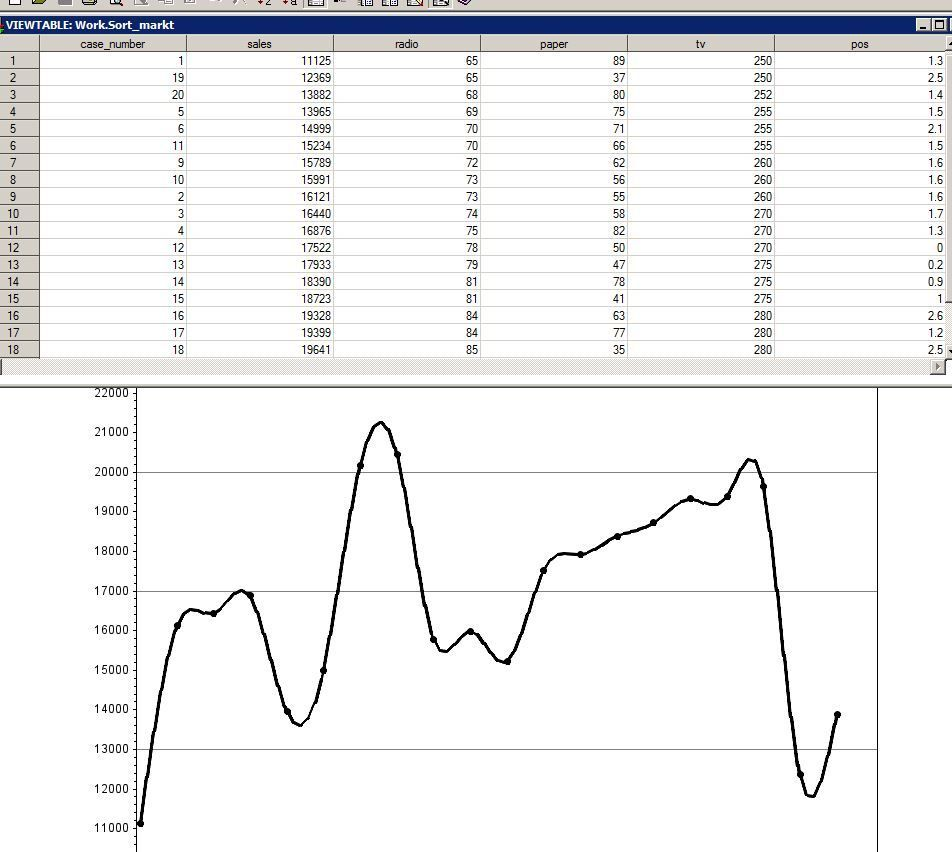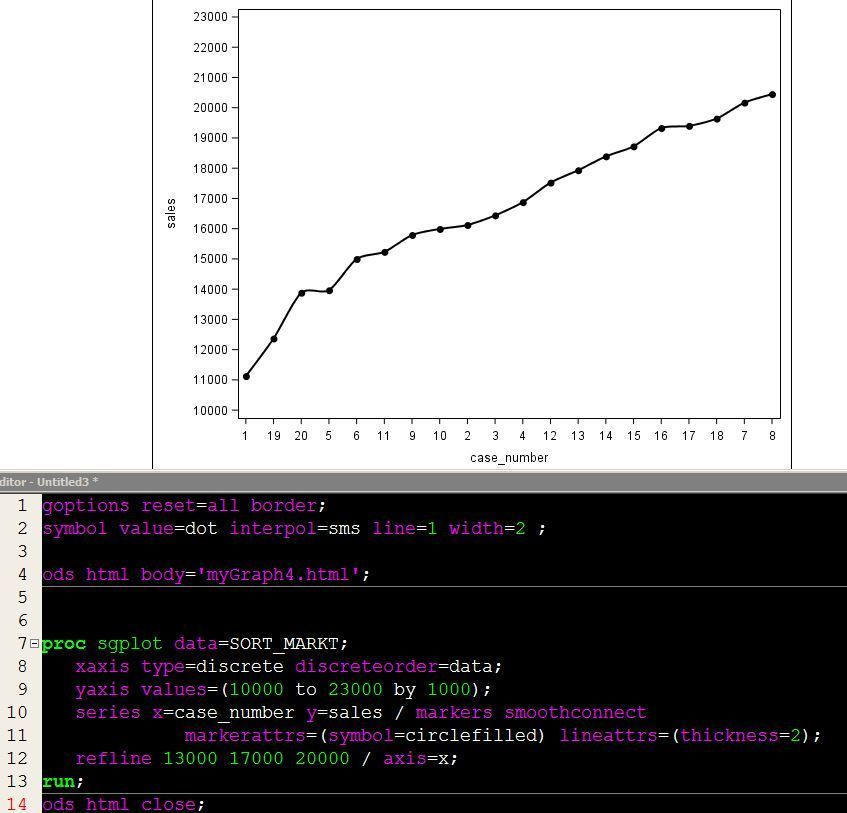## Sort is not being displayed when running PROC GPLOT statement

Hello!

I've sorted my data and created a sorted output data set ("SORT_MARKT"). I'm attempting to use that sorted data set to plot my data and show an ascending trend line, but once the data is plotted, it's displayed as if no sort was ever applied. I'm not sure what exactly is causing this to display this way.

My code:

PROC SORT DATA = MARKETING OUT=SORT_MARKT ;
BY sales  ;
RUN;

goptions reset=all border;
symbol value=dot interpol=sms line=1 width=2 ;
proc gplot data=SORT_MARKT ;
plot sales*case_number /  haxis = 1 to 21 by 1
vaxis = 10000 to 23000 by 1000
vref = 13000 17000 20000    ;
run;
quit;

The attached picture shows the sorted data set and the proc gplot output. What am I missing?1 ACCEPTED SOLUTION

Accepted SolutionsDanH_sas
SAS Super FREQ

## Re: Sort is not being displayed when running PROC GPLOT statement

PROC GPLOT does not support data order, unless you use one of the "client" devices (actximg, activex, javaimg, java). If you want to try that approach, you will also need to use the DATAORDER option on the PLOT statment. However, I would recommend that you use SGPLOT instead to create this plot:

``````proc sgplot data=SORT_MARKT;
xaxis type=discrete discreteorder=data;
yaxis values=(10000 to 23000 by 1000);
series x=sales y=case_number / markers smoothconnect
markerattrs=(symbol=circlefilled) lineattrs=(thickness=2);
refline 13000 17000 20000 / axis=x;
run;
``````

Hope this helps!

Dan

2 REPLIES 2DanH_sas
SAS Super FREQ

## Re: Sort is not being displayed when running PROC GPLOT statement

PROC GPLOT does not support data order, unless you use one of the "client" devices (actximg, activex, javaimg, java). If you want to try that approach, you will also need to use the DATAORDER option on the PLOT statment. However, I would recommend that you use SGPLOT instead to create this plot:

``````proc sgplot data=SORT_MARKT;
xaxis type=discrete discreteorder=data;
yaxis values=(10000 to 23000 by 1000);
series x=sales y=case_number / markers smoothconnect
markerattrs=(symbol=circlefilled) lineattrs=(thickness=2);
refline 13000 17000 20000 / axis=x;
run;
``````

Hope this helps!

Dan

## Re: Sort is not being displayed when running PROC GPLOT statement

Thanks, Dan. I finally saw what you meant about the PROC GPLOT route and opted to go with SGPLOT. Made one minor tweak to the Series statement.